# Basic data analysis with palmerpenguins

Interested in publishing a one-time post on R-bloggers.com? Press here to learn how.``` ```

Introduction

In June 17, nice article for introducing new trial dataset were uploaded via R-bloggers.

iris, one of commonly used dataset for simple data analysis. but there is a little issue for using it.

Too good.

Every data has well-structured and most of analysis method works with iris very well.

In reality, most of dataset is not pretty and requires a lot of pre-process to just start. This can be possible works in pre-process

Remove `NA`s.
Select meaningful features
Handle `duplicated` or `inconsistent` values.
or even, just `loading` the dataset. if is not well-structured like Flipkart-products

However, in this penguin dataset, you can try for this work. also there’s pre-processed data too.

There is a routine for me with brief data analysis. and today, I want to share them with this lovely penguins.

Contents

0. Load dataset and library on workspace.
```library(palmerpenguins) # for data
library(dplyr) # for data-handling
library(corrplot) # for correlation plot
library(GGally) # for parallel coordinate plot
library(e1071) # for svm

data(penguins) # load pre-processed penguins ```
`palmerpenguins` have 2 data `penguins`, `penguins_raw` , and as you can see from their name, `penguins` is pre-processed data.

1. See the `summary`  and `plot` of Dataset
```summary(penguins)
plot(penguins)
```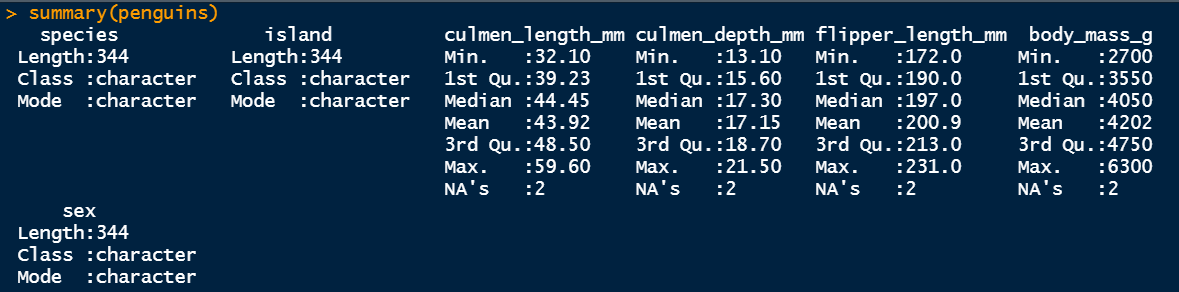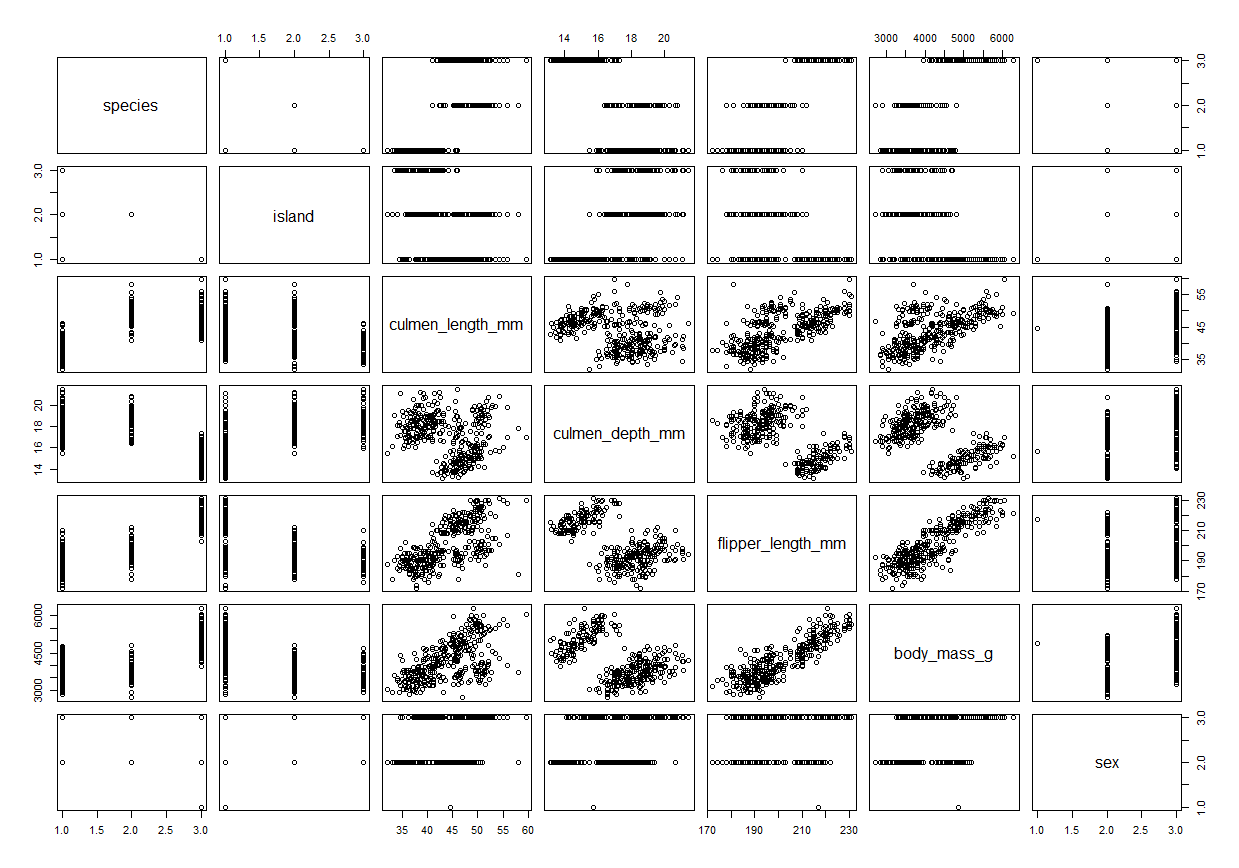It seems `species``island`  and `sex` is categorical features.
and remaining for numerical features.

2. Set the format of feature
```penguins\$species <- as.factor(penguins\$species)
penguins\$island <- as.factor(penguins\$island)
penguins\$sex <- as.factor(penguins\$sex)

summary(penguins)
plot(penguins)
```
and see `summary` and `plot` again. note that result of `plot` is same.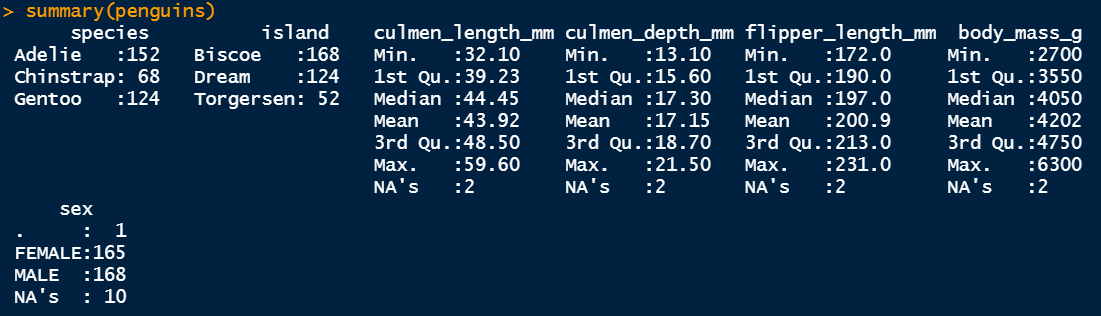There’s unwanted `NA` and `.` values in some features.

3. Remove not necessary datas ( in this tutorial, `NA`)
```penguins <- penguins %>% filter(sex == 'MALE' | sex == 'FEMALE')
summary(penguins)
```
And here, I additionally defined color values for each penguins to see better `plot` result
```# Green, Orange, Purple
pCol <- c('#057076', '#ff8301', '#bf5ccb')
plot(penguins, col = pCol[penguins\$species], pch = 19)
```Now, plot results are much better to give insights.

Note that, other pre-process step may requires for different datasets.

4. See relation of categorical features

My first purpose of analysis this penguin is `species`
So, I will try to see relation between `species` and other categorical values

4-1. `species`, `island`
```table(penguins\$species, penguins\$island)
chisq.test(table(penguins\$species, penguins\$island)) # meaningful difference

ggplot(penguins, aes(x = island, y = species, color = species)) +
geom_jitter(size = 3) +
scale_color_manual(values = pCol)

```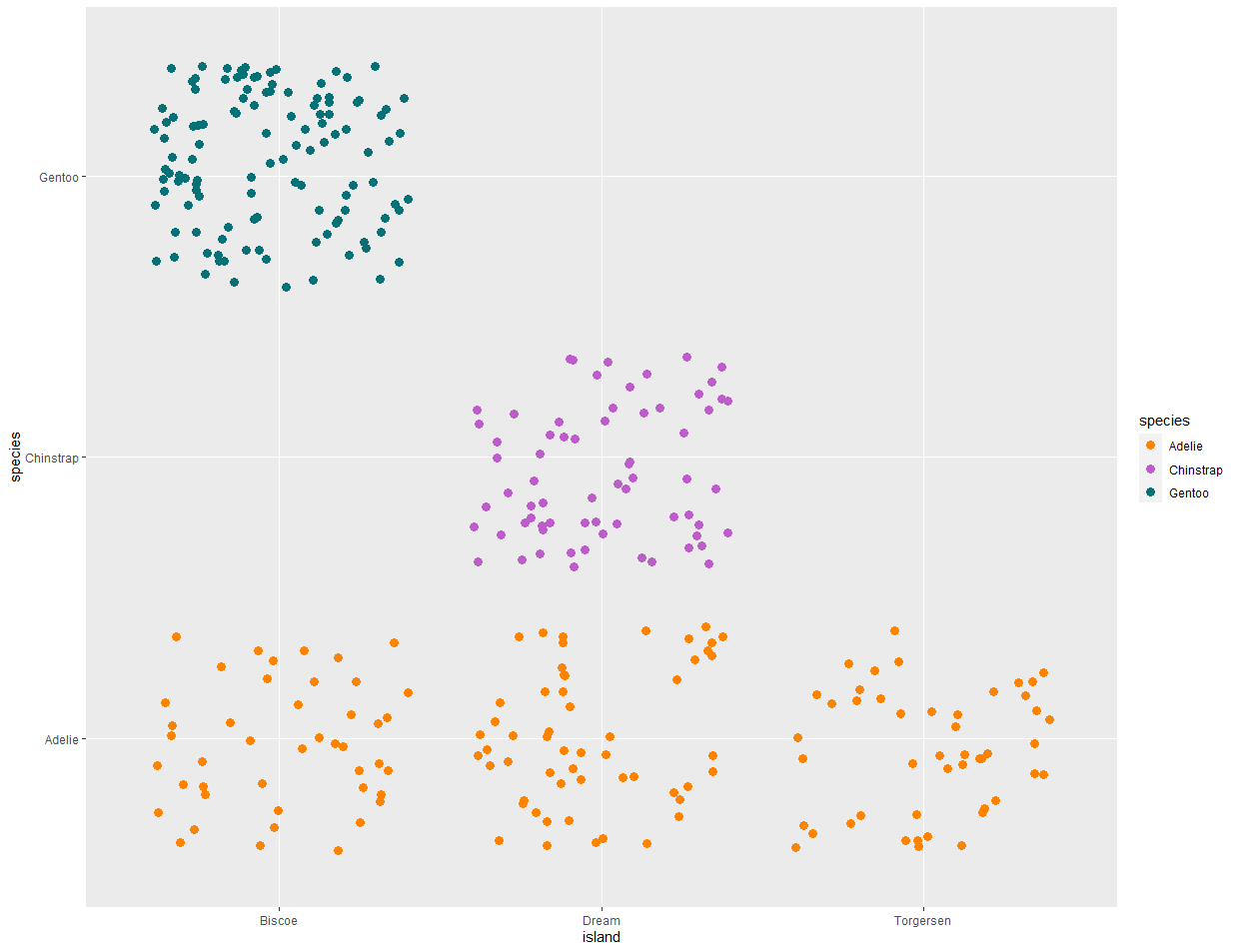Wow, there’s strong relationship between `species` and `island`

`Adelie` lives in every island
`Gentoo` lives in only `Biscoe`
`Chinstrap` lives in only `Dream`

4-2 & 4.3.
However, `species` and `sex` or `sex` and `island` did not show any meaningful relation.
You can try following codes.
```# species vs sex
table(penguins\$sex, penguins\$species)
chisq.test(table(penguins\$sex, penguins\$species)[-1,]) # not meaningful difference 0.916

# sex vs island
table(penguins\$sex, penguins\$island) # 0.9716
chisq.test(table(penguins\$sex, penguins\$island)[-1,]) # not meaningful difference 0.9716
```
5. See with numerical features

I will select numerical features.
and see correlation plot and parallel coordinate plots.
```# Select numericals
penNumeric <- penguins %>% select(-species, -island, -sex)

# Cor-relation between numerics

corrplot(cor(penNumeric), type = 'lower', diag = FALSE)

# parallel coordinate plots

ggparcoord(penguins, columns = 3:6, groupColumn = 1, order = c(4,3,5,6)) +
scale_color_manual(values = pCol)

plot(penNumeric, col = pCol[penguins\$species], pch = 19)

```
and below are result of them.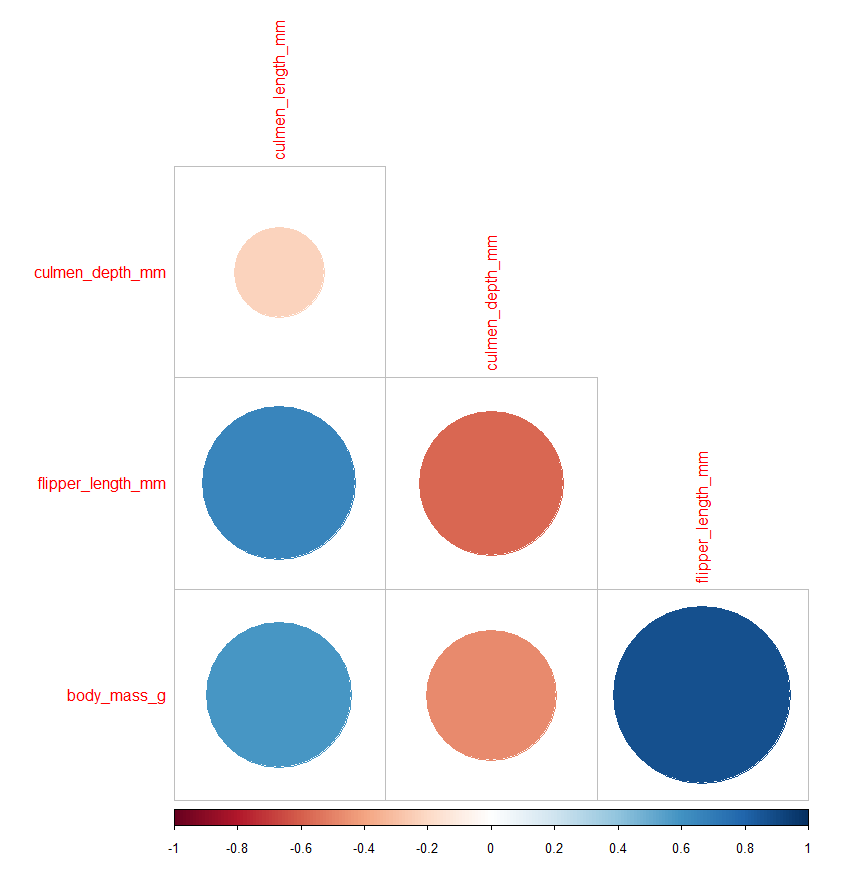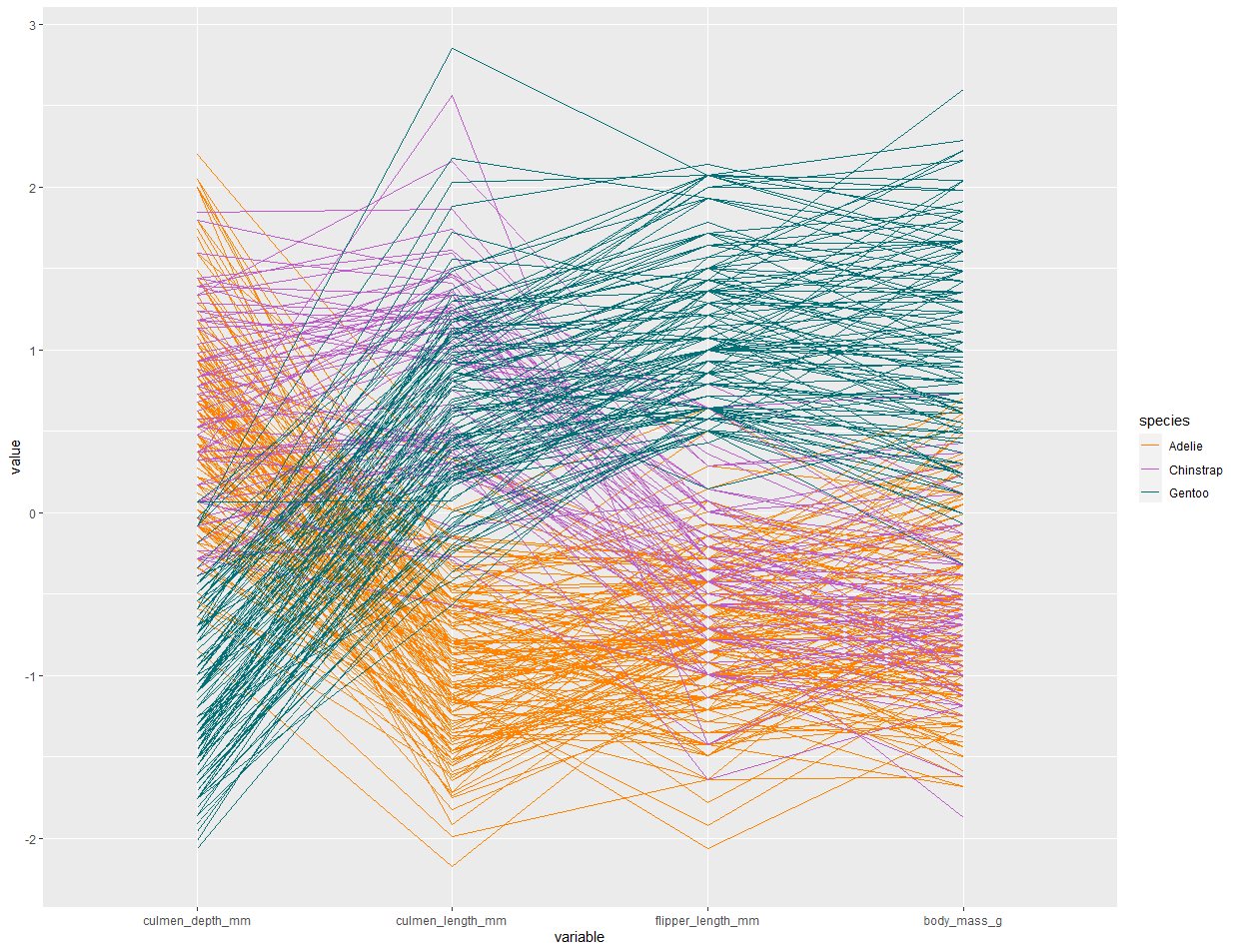lucky, every numeric features (even only 4) have meaningful correlation and there is trend with  their combination for `species` (See parallel coordinate plot)

6. Give statistical work on dataset.

In this step, I usually do `linear modeling` or `svm` to predict

6.1 `linear modeling`

`species` is categorical value, so it needs to be change to numeric value
```set.seed(1234)
idx <- sample(1:nrow(penguins), size = nrow(penguins)/2)

# as. numeric
speciesN <- as.numeric(penguins\$species)
penguins\$speciesN <- speciesN

train <- penguins[idx,]
test <- penguins[-idx,]

fm <- lm(speciesN ~ flipper_length_mm + culmen_length_mm + culmen_depth_mm + body_mass_g, train)

summary(fm)
```It shows that, `body_mass_g` is not meaningful feature as seen in `plot` above ( it may explain `gentoo`, but not other penguins )

To predict, I used this code. however, numeric predict generate not complete value (like 2.123 instead of 2) so I added rounding step.
```predRes <- round(predict(fm, test))
predRes[which(predRes>3)] <- 3
predRes <- sort(names(pCol))[predRes]

test\$predRes <- predRes
ggplot(test, aes(x = species, y = predRes, color = species))+
geom_jitter(size = 3) +
scale_color_manual(values = pCol)

table(test\$predRes, test\$species)
```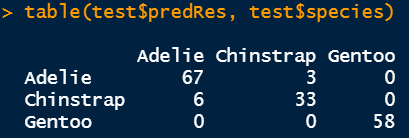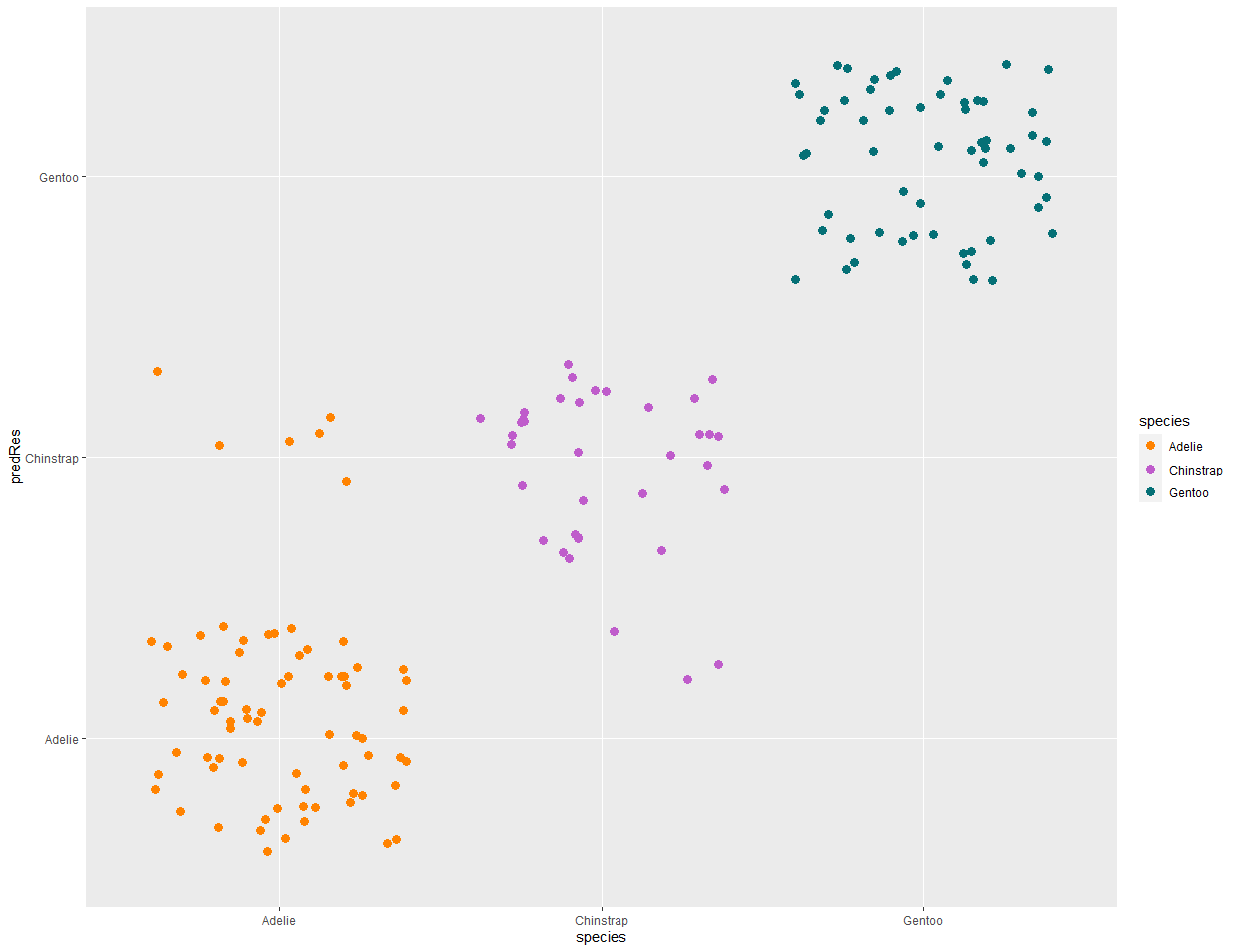Accuracy of basic `linear modeling` is 94.6%

6-2 `svm`

using `svm` is also easy step.
```m <- svm(species ~., train)

predRes2 <- predict(m, test)
test\$predRes2 <- predRes2

ggplot(test, aes(x = species, y = predRes2, color = species)) +
geom_jitter(size = 3) +
scale_color_manual(values = pCol)

table(test\$species, test\$predRes2)
```
and below are result of this code.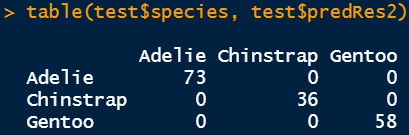Accuracy of `svm` is 100%. wow.

Conclusion

Today I introduced simple routine for EDA and statistical analysis with penguins.
That is not difficult that much, and shows good performances.

Of course, I skipped a lot of things like processing raw-dataset.
However I hope this trial gives inspiration for further data analysis.

Thanks.

## One thought on “Basic data analysis with palmerpenguins”

This site uses Akismet to reduce spam. Learn how your comment data is processed.